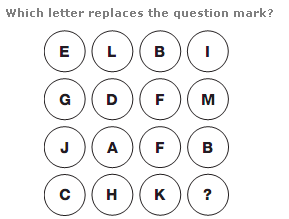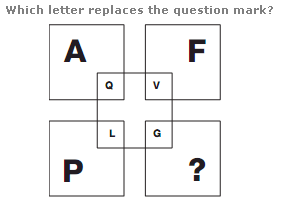# Puzzles - Missing letters puzzles

### Exercise :: Missing letters puzzlesAnswer : A Explanation : In each column of the diagram, the sum of the numerical values of the letters is always 25.Answer : W Explanation : In each group of 3 squares, starting on the upper left and moving clockwise around the other 2 squares, letters follow the alphabetic sequence in steps of 6 for the left hand diagram, 7 for the upper middle, 8 for the right hand diagram and 9 for the lower one.Answer : K Explanation : Add 6 to the numerical value of each of the larger letters, and write the letter with this value in the smaller square diagonally opposite.